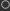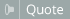Thread: Recieve from serial View Single Post01-27-2019, 02:32 PM #6 Kaitain Human being with feelings   Join Date: Nov 2016 Posts: 34Some progress here. What I'm trying to achieve is to send a float number fom my MIDI controller (Teensy) to OSCiiBot. This float number is normalized to 1024, so it has decimals and it goes from 0 to 1. So far I've manged to pack this float into a SysEx message, like this: for (int i = 0; i <= 3; i++) { paramValMSB[i] = (mapped.bytes[i] >> 4) & B00001111; paramValLSB[i] = mapped.bytes[i] & B00001111; } // SysEx message assemby uint8_t buff[] = {SysExStart, paramNumMSB, paramNumLSB, paramValMSB, paramValLSB, paramValMSB, paramValLSB, paramValMSB, paramValLSB, paramValMSB, paramValLSB, SysExEnd}; // Sens SysEx UsbMIDI.sendSysEx(sizeof(buff), buff, true); A float has 4 bytes. And I had to split evey one of the 4 bytes of the float variable (paramVal) into 2 bytes (MSB and LSB), in order to have the bit 7 set to 0 on each byte, according to the MIDI protocol. Although this is not a very efficient solution it works. Now, in OSCii Bot I wrote this to receive and reconstruct each byte: valByte0 = ((str_getchar(oscstr, 3) << 4) & 0xF0) | str_getchar(oscstr, 4); valByte1 = ((str_getchar(oscstr, 5) << 4) & 0xF0) | str_getchar(oscstr, 6); valByte2 = ((str_getchar(oscstr, 7) << 4) & 0xF0) | str_getchar(oscstr, 8); valByte3 = ((str_getchar(oscstr, 9) << 4) & 0xF0) | str_getchar(oscstr, 10); Checking every one the bytes received, they match byte per byte with the ones sent from the controller. So far so good. Now, the problem is how to construct a float number with these four bytes. I tryed this: val = ((valByte3 << 24) & 0xFF000000) | ((valByte2 << 16) & 0xFF0000) | ((valByte1 << 8) & 0xFF00) | valByte0; And although I get a 4 byte value with the 4 bytes in their right position and order, the value is interpreted as an integer. It doesn't follow the IEEE protocol: taking some bits for the sing, some for the exponent and some for the mantisa (which are indeed observed at the Arduino IDE on the other end). This is something I don't understand because inthe OSCii bot code reference says "Variables do not need to be declared, are by default global, and are all double-precision floating point". So my question is this: how can I construct a float value in OSCii bot starting from 4 bytes? Is there any chance to make some casting like "float(var)" or something? (I tried, didn´t work). Sorry for the very long post, but I couldn`t find a sorter way to explain this